# Our case

Our case is to study changing the sphere of usage verbs to ‘mignut’ (to blink) and ‘podmignut’(to wink). Material for analysis we found at the Corpus of texts of the XIX century, collected in the framework of the “Taman Today” project, namely: the marked novel by M.Yu. Lermontov “The hero of Our Time”, 1840

In the chapter of the novel “Hero of Our Time” “Princess Mary” we found the following examples

• Что же? - сказал пьяный господин, мигнув драгунскому капитану, который ободрял его знаками, - разве вам не угодно?..

Капитан мигнул Грушницкому, и этот, думая, что я трушу, принял гордый вид, хотя до сей минуты тусклая бледность покрывала его щеки

According to the Small Academic Dictionary (МАС), the verb ‘migat’ has 3 meanings

1. To move quickly down and raise the eyelids and eyelashes; blink
2. To sign by the movement of the eyelids
3. To glow with a weak, uneven, wavering light (about luminous and luminous objects); Twinkle

# Our data

We used data from the National Corpora of Russian language. For the verb ‘mignut’, we found 877 occurrences in the Corpora, 35% were chosen by random sampling. The received entries were classified by the values: 1-‘to blink’, 2 –‘to sign’, 3 – ‘to twinkle’. There are 120 occurrences of considering meaning ‘to sign’ (2).

For the verb ‘podmignut’, we found 2372 occurrences in the Corpora, 35% were chosen by random sampling and we got 830 occurrences for further analysis.

# Hypothesis

The null hypothesis: Verbs with prefixes appear later, displacing no prefixes equivalents.
The alternative hypothesis: There is no correlation between these two verbs.

# First look at the data

mignut <- read.csv("мигнуть 1.csv", sep=";")
podmignut <- read.csv("подмигнуть 1.csv", sep=";")

Also we have introduced the new field ‘category’. It is a numeric value which takes value 1 if it’s a verb without prefix ‘под’ and 2 in opposite case.

mignut$category = 1 podmignut$category = 2

Once we loaded the data, we tried to normalize it. We considered division of number of entries in data frame from each year on total numer of entries as normalization.

count_mignut <- nrow(mignut[mignut$meaning == 2, ]) count_podmignut <- nrow(podmignut) mignut_init_distr <- mignut[mignut$meaning == 2, ] %>%
group_by(Publ_year, category) %>%
summarise(dist_per = n() / count_mignut)

podmignut_init_distr <- podmignut %>%
group_by(Publ_year, category) %>%
summarise(dist_per = n() / count_podmignut)

Now we tried to see the diagrams which can show the tendencies of usage of these verbs to make some empirical conclusions. Our distributions are:

merged_distr_sep <- rbind(mignut_init_distr, podmignut_init_distr)

merged_distr_sep %>%
ggplot(aes(Publ_year, dist_per))+
geom_smooth()+
facet_wrap(~category)
## geom_smooth() using method = 'loess'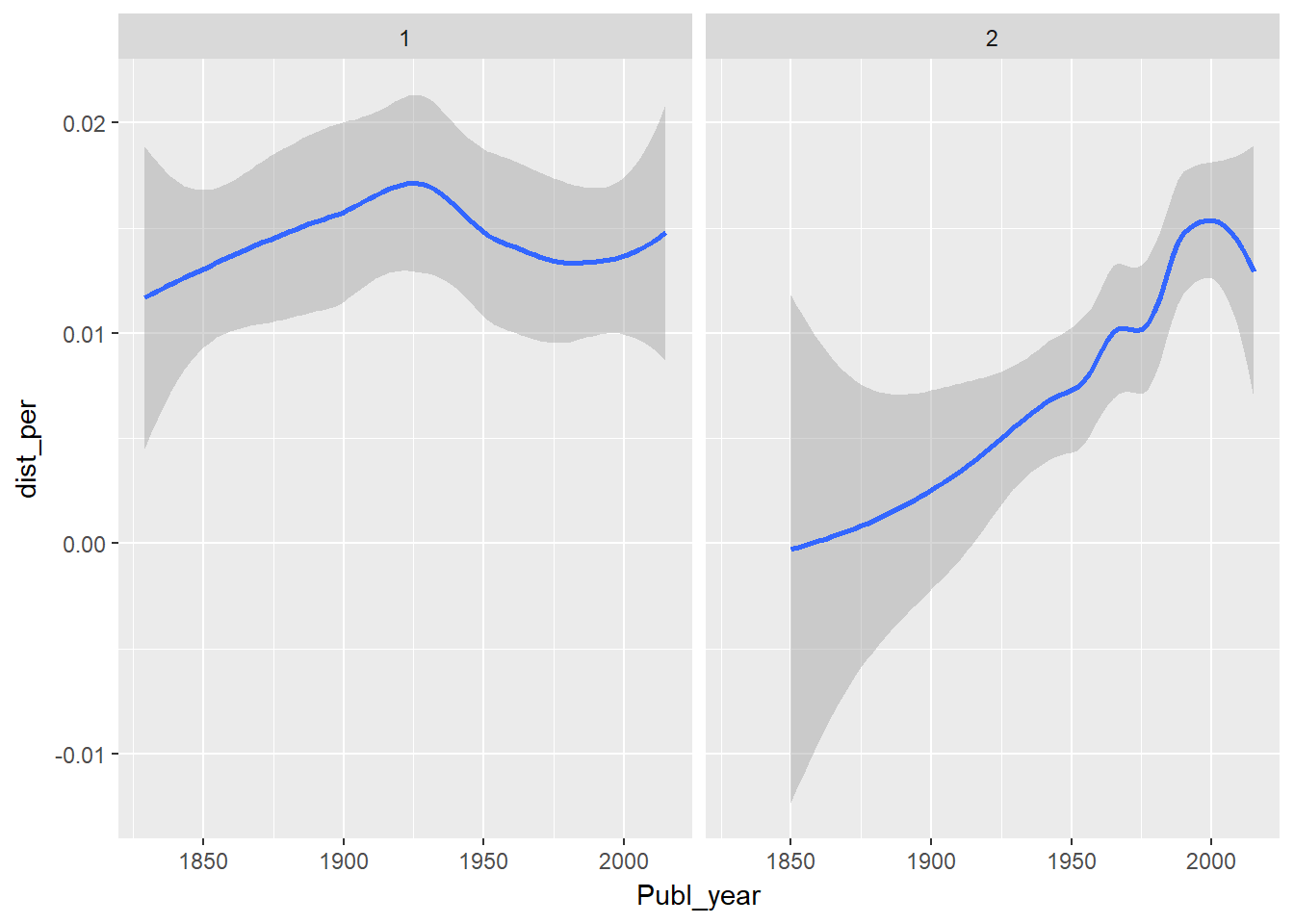Taking first look on these diagrams we can observe that our hypothesis actually tends to be true. We should take into account that we have much less texts in 1850’s than now. So, it’s quite logical that we have such low values in that period. On the other hand, the peak of ‘мигнуть’ usage is in 1920’s, but the peak of ‘подмигнуть’ usage is in 1990’s. This fact lets us say that ‘подмигнуть’ tends to replace the ‘мигнуть’.

But these are just some emprircal conclusions. Next thing that we did is applying some statistical tests to our data

# Preprocessing the data

Before we start applying the statistical tests, we have to preprocess our data, as we mentioned. For adequate results of statistical tests, it is necessary to compare comparable data sets, in our particular case it is necessary to select a time interval beginning with one date for both verbs ‘mignut’ and ‘podmignut’.

mignut <- read.csv("D:\\материалы\\мигнуть 1.csv", sep=";")
podmignut <- read.csv("D:\\материалы\\подмигнуть 1.csv", sep=";")

podmignut.1=sample(podmignut$Position,0.35*nrow(podmignut)) podmignut=podmignut[podmignut.1,] par(mfrow=c(1,2)) hist(mignut[mignut$meaning==2,]$Publ_year,freq=F) hist(podmignut$Publ_year,freq=F)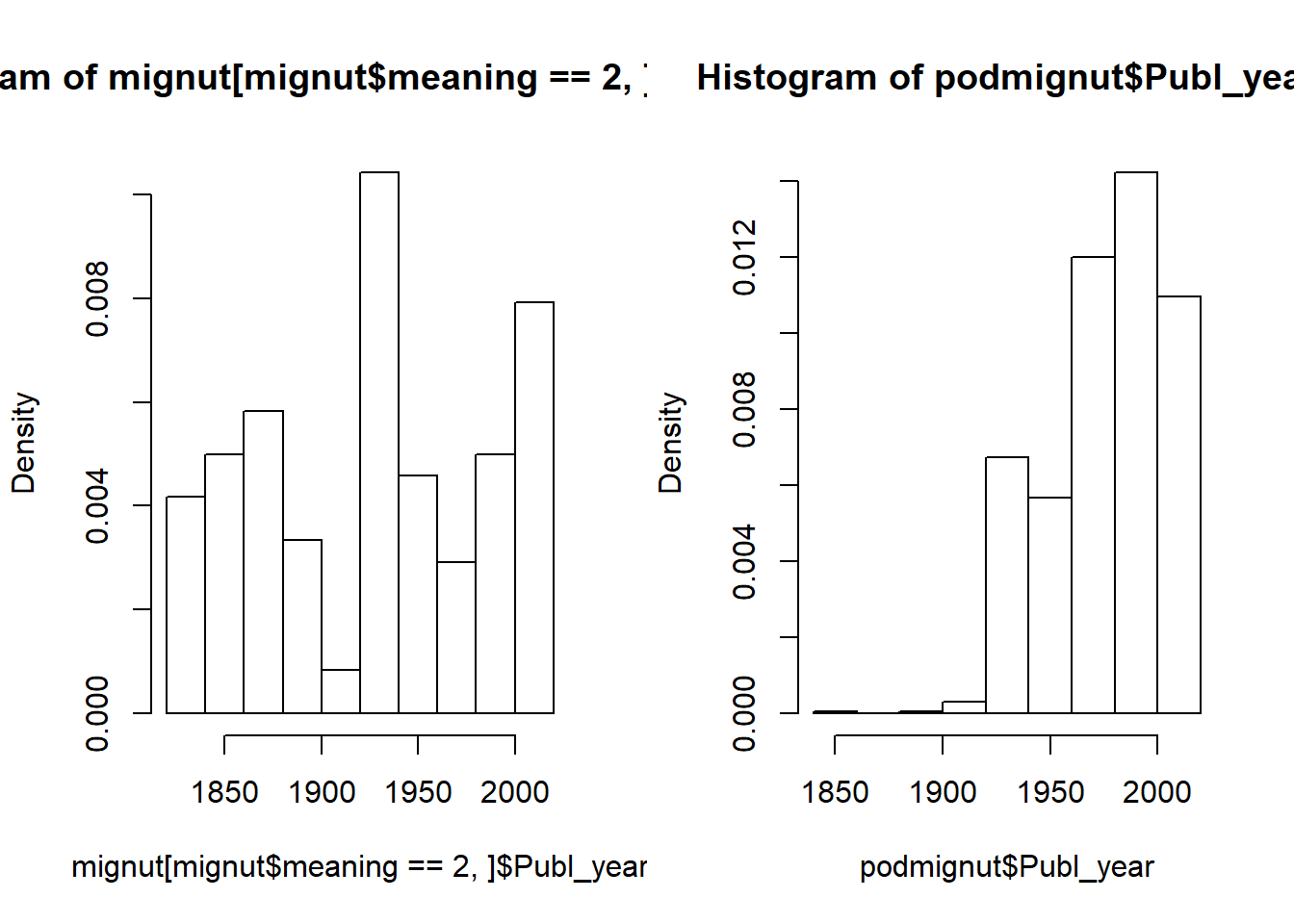mignut=mignut[mignut$Publ_year>min(podmignut$Publ_year),]

mignut$category = 1 podmignut$category = 2

count_mignut <- nrow(mignut[mignut$meaning == 2, ]) count_podmignut <- nrow(podmignut) mignut_init_distr <- mignut[mignut$meaning == 2, ] %>%
group_by(Publ_year, category) %>%
summarise(dist_per = n() / count_mignut)

podmignut_init_distr <- podmignut %>%
group_by(Publ_year, category) %>%
summarise(dist_per = n() / count_podmignut)

merged_distr_sep <- rbind(mignut_init_distr, podmignut_init_distr)

merged_distr_sep %>%
ggplot(aes(Publ_year, dist_per))+
geom_smooth()+
facet_wrap(~category)
## geom_smooth() using method = 'loess'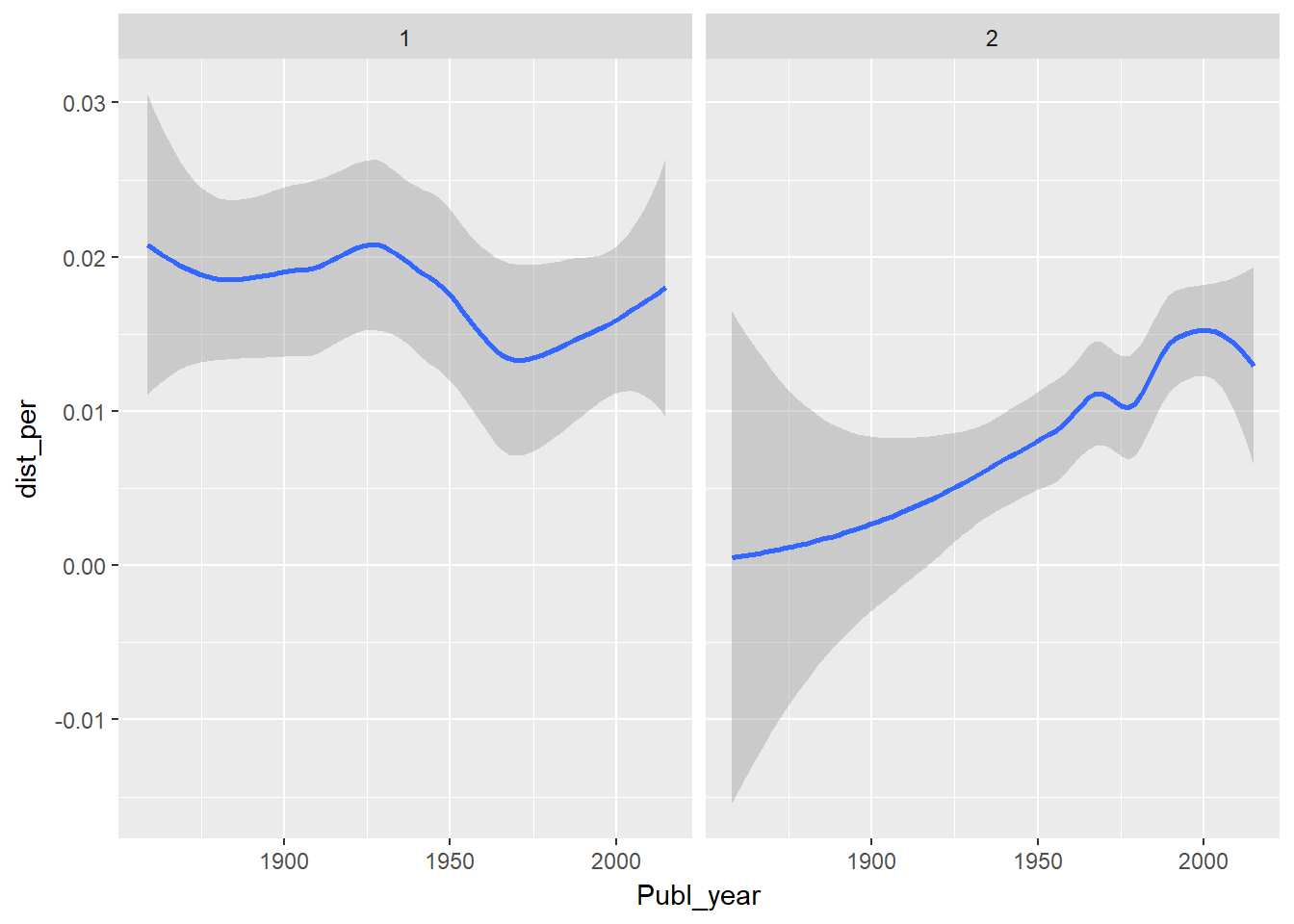# Applying statistical methods

Data can be either ranged or distributed. The distributed data refers to random values from some continuous sets. Ranged data refers to some categories. Distributed values use t-test for hypothesis demonstration, but ranged ones use chi-squared tests.

We have two distributed values, that’s why we have used paired t-test:

merged_distr_2 <- merge(x = mignut_init_distr, y = podmignut_init_distr,
by = "Publ_year", all = TRUE) [c(1, 3, 5)]
t.test(merged_distr_2$dist_per.x, merged_distr_2$dist_per.y, paired = TRUE)
##
##  Paired t-test
##
## data:  merged_distr_2$dist_per.x and merged_distr_2$dist_per.y
## t = 2.1605, df = 39, p-value = 0.03694
## alternative hypothesis: true difference in means is not equal to 0
## 95 percent confidence interval:
##  0.0003524832 0.0106999619
## sample estimates:
## mean of the differences
##             0.005526223

The obtained p-value is enough to proove our hupothesis, but let’s think about our data in another way. Now let’s talk about our values from point of view of the time series.

# Time series

We have used the ‘ts’ function to process our data as time series:

mignut_init_distr2 <- data.frame(Publ_year = c(min(mignut_init_distr$Publ_year): max(mignut_init_distr$Publ_year)),
category = 1,
dist_per = 0)

mignut_init_distr2[mignut_init_distr2$Publ_year %in% mignut_init_distr$Publ_year,] <-
mignut_init_distr

mignut_time_serie <- ts(mignut_init_distr2$dist_per, start = min(mignut_init_distr2$Publ_year))

podmignut_init_distr2 <- data.frame(Publ_year = c(min(podmignut_init_distr$Publ_year): max(podmignut_init_distr$Publ_year)),
category = 1,
dist_per = 0)

podmignut_init_distr2[podmignut_init_distr2$Publ_year %in% podmignut_init_distr$Publ_year,] <-
podmignut_init_distr

podmignut_time_serie <- ts(podmignut_init_distr2$dist_per, start = min(podmignut_init_distr2$Publ_year))

Now we can watch its diagram:

plot.ts(mignut_time_serie, type = "l")
lines(lowess(mignut_time_serie), col = "red", lty = "dashed")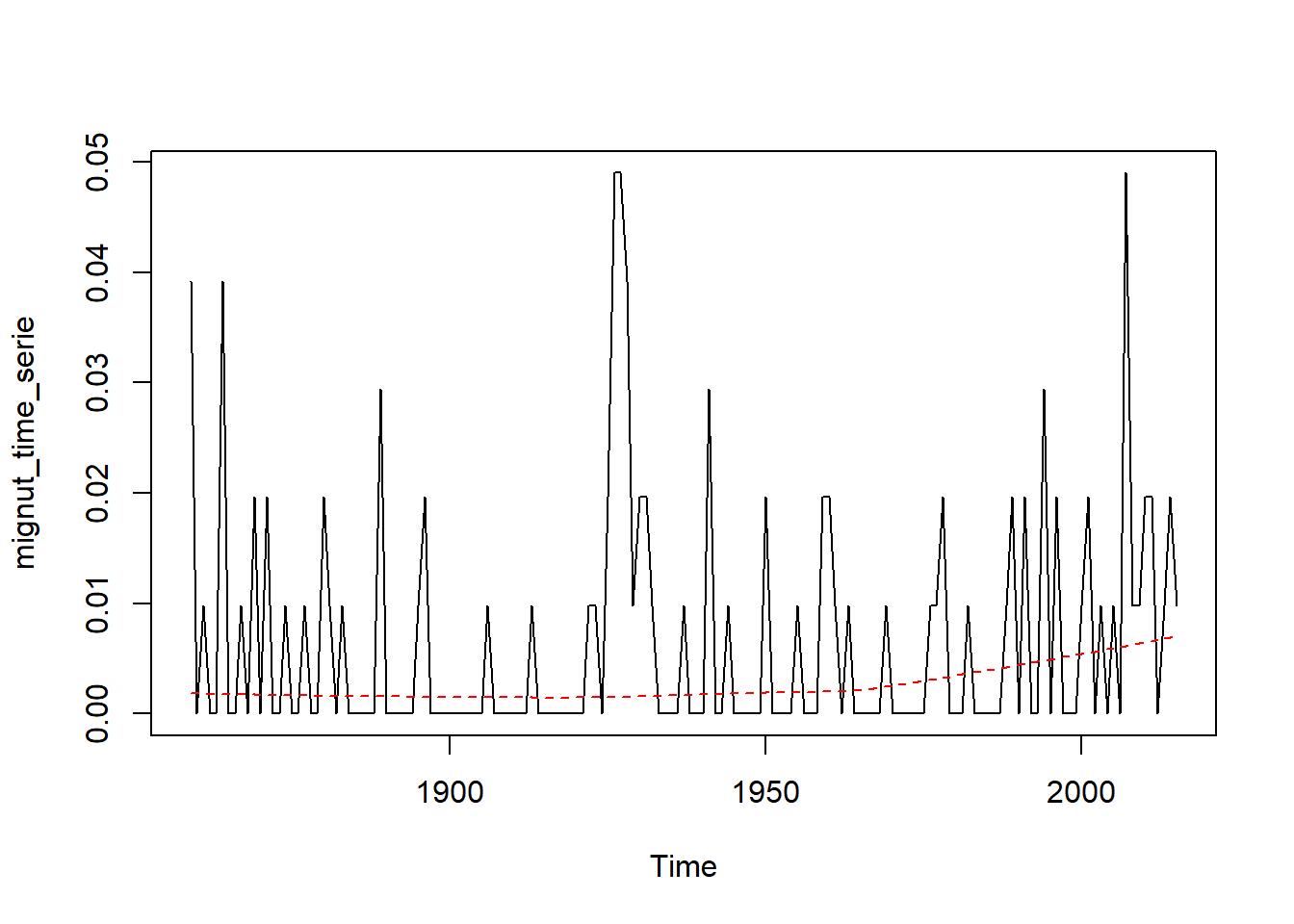plot.ts(podmignut_time_serie, type = "l")
lines(lowess(podmignut_time_serie), col = "red", lty = "dashed")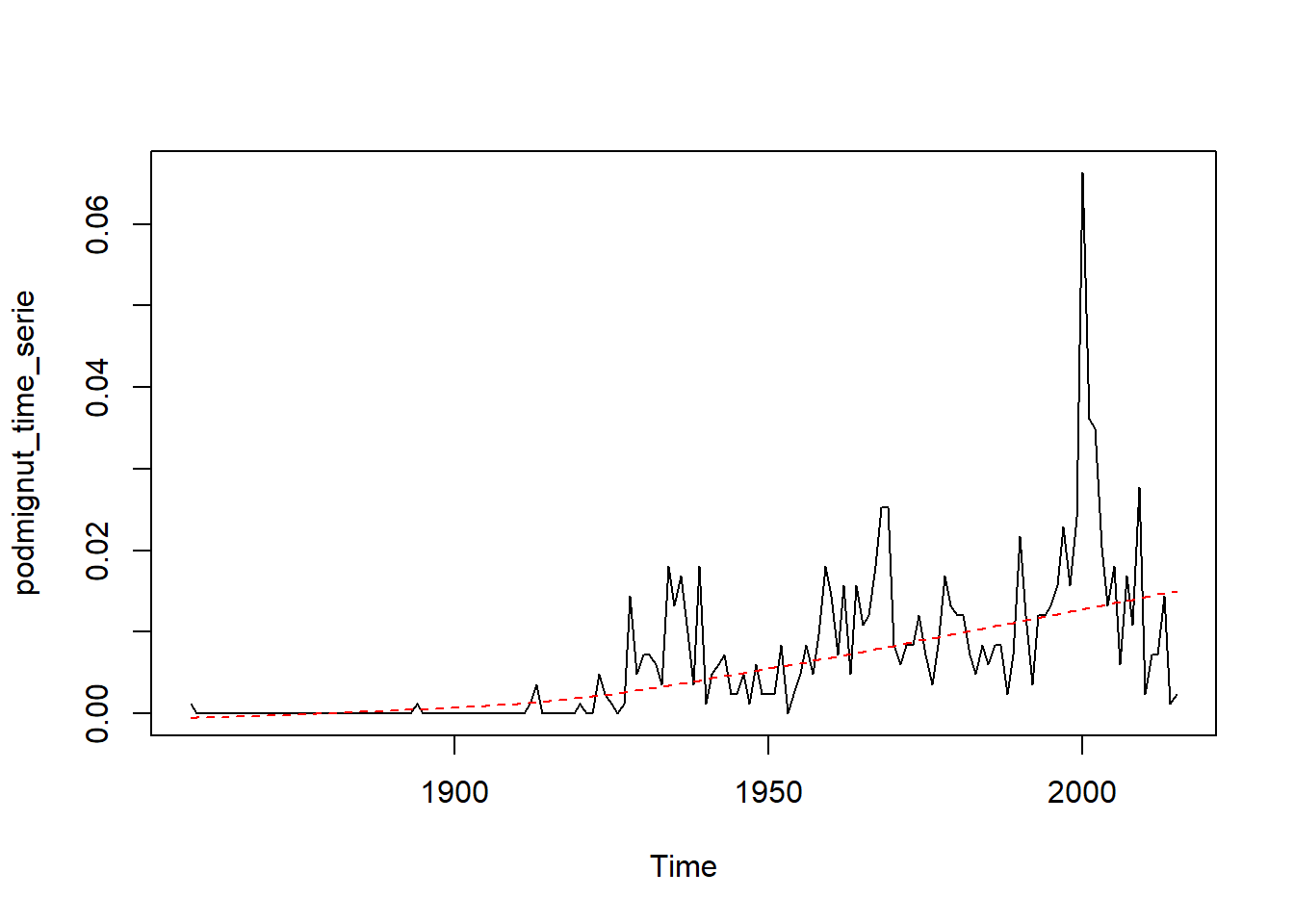Next we’ve used SMA algorithm of smoothing our data

mignut_time_serie_sma <- SMA(mignut_time_serie, n = 3)
plot.ts(mignut_time_serie, type = "l")
lines(lowess(mignut_time_serie_sma), col = "red", lty = "dashed")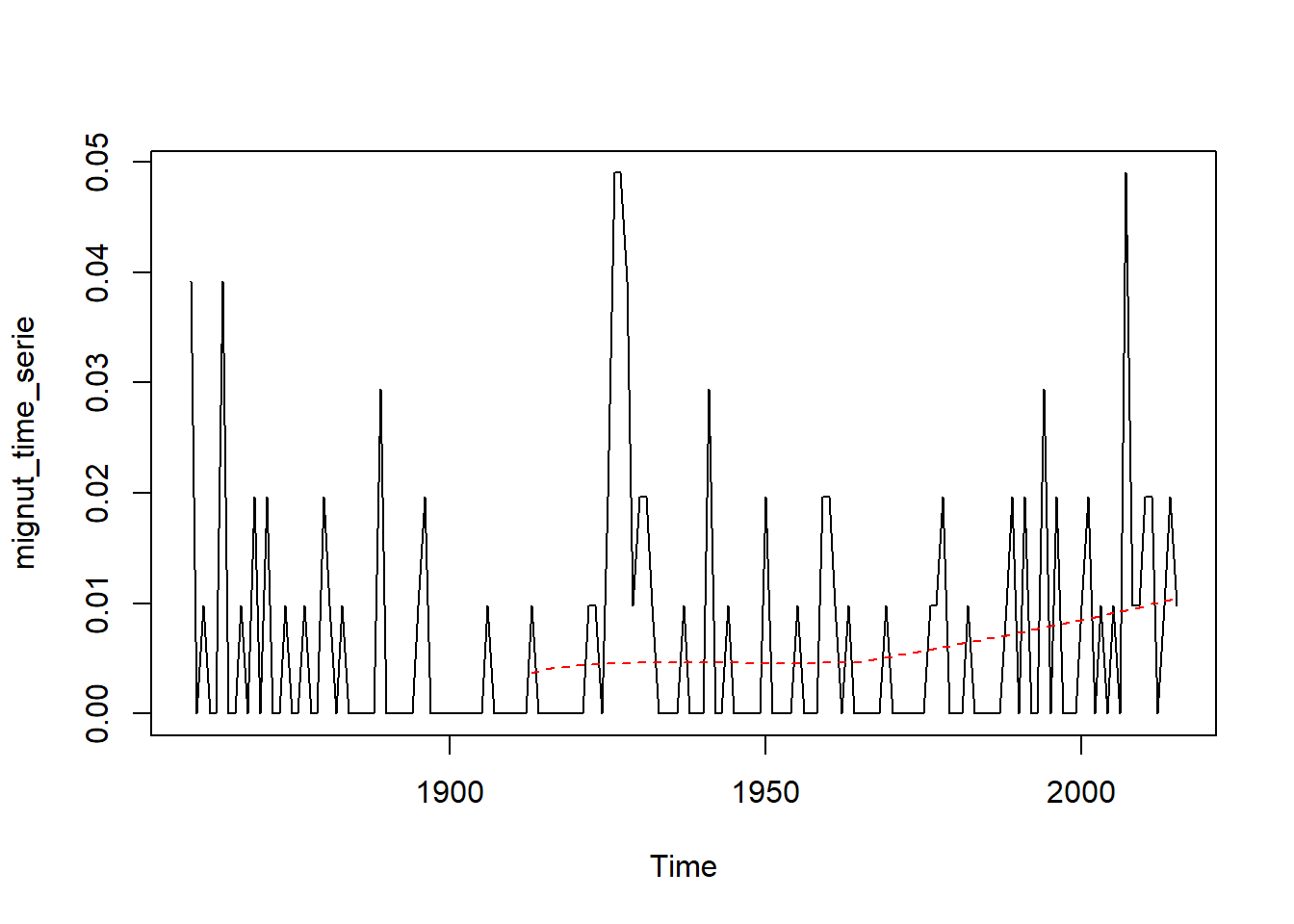podmignut_time_serie_sma <- SMA(podmignut_time_serie, n = 3)
plot.ts(podmignut_time_serie, type = "l")
lines(lowess(podmignut_time_serie_sma), col = "red", lty = "dashed")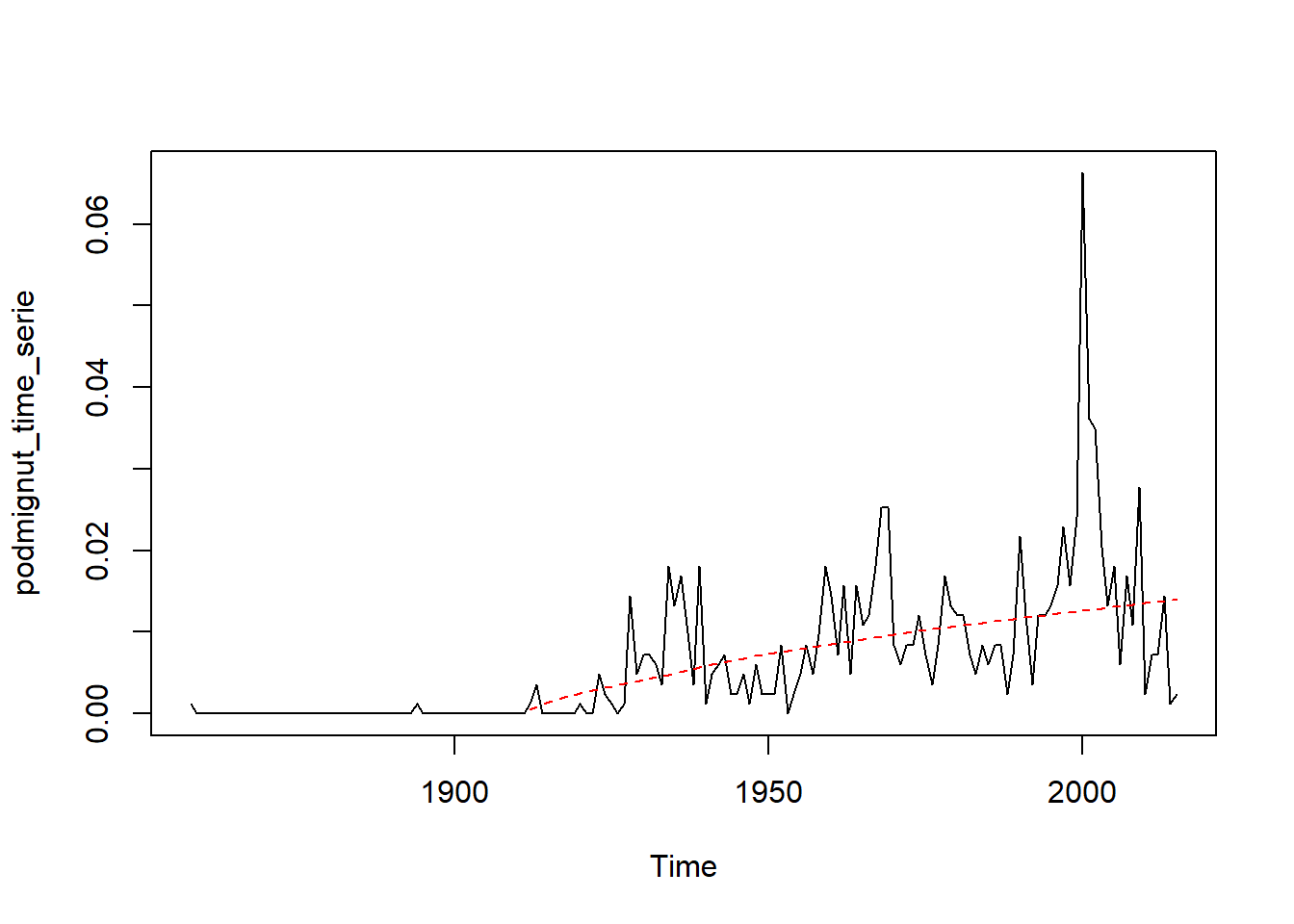According to this diagrams we can say that count of text in last time has been increased, that’s why count of ‘mignut’ increases too. But in the case of ‘podmignut’ there’s another thing. Its number increases constantly.

adf.test(diff(mignut_time_serie), alternative = c("stationary"))
## Warning in adf.test(diff(mignut_time_serie), alternative =
## c("stationary")): p-value smaller than printed p-value
##
##  Augmented Dickey-Fuller Test
##
## data:  diff(mignut_time_serie)
## Dickey-Fuller = -7.3695, Lag order = 5, p-value = 0.01
## alternative hypothesis: stationary
adf.test(diff(podmignut_time_serie), alternative = c("stationary"))
## Warning in adf.test(diff(podmignut_time_serie), alternative =
## c("stationary")): p-value smaller than printed p-value
##
##  Augmented Dickey-Fuller Test
##
## data:  diff(podmignut_time_serie)
## Dickey-Fuller = -6.9831, Lag order = 5, p-value = 0.01
## alternative hypothesis: stationary

Now we wanted to show that our data is not stationary, so we can do some forecasts

Now let’s see on Holt - Winters forecasts:

HoltWinters(mignut_time_serie, beta = FALSE, gamma = FALSE)
## Holt-Winters exponential smoothing without trend and without seasonal component.
##
## Call:
## HoltWinters(x = mignut_time_serie, beta = FALSE, gamma = FALSE)
##
## Smoothing parameters:
##  alpha: 0.3171628
##  beta : FALSE
##  gamma: FALSE
##
## Coefficients:
##         [,1]
## a 0.01249528
HoltWinters(podmignut_time_serie, beta = FALSE, gamma = FALSE)
## Holt-Winters exponential smoothing without trend and without seasonal component.
##
## Call:
## HoltWinters(x = podmignut_time_serie, beta = FALSE, gamma = FALSE)
##
## Smoothing parameters:
##  alpha: 0.4528763
##  beta : FALSE
##  gamma: FALSE
##
## Coefficients:
##         [,1]
## a 0.00476366

The most important thing here is that the mignut series forecast alpha parameter is closer to 0 than podmignut one. This means that mignut prediction is mostly based on the late time period (when number of texts is bigger).

# Results

As a result, we have received confirmation of our hypothesis, however, the database for an ideal statistical analysis is not enough, but for existing occurrences we have observed some significant correlation.